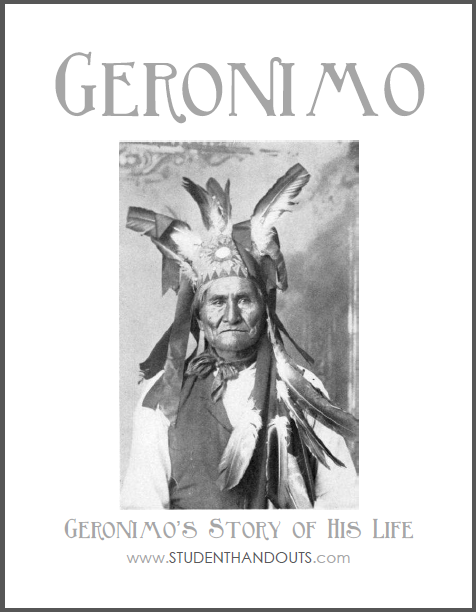Geronimo's Story of His Life - Workbook | Student Handouts

Geronimo's Story of His Life+ + B R + N L S + C N + + + R + F S + O
+ O + + I D O L O O A + + + A + O E + M
W + + + E F + Y I U Y V + + I + R L + I
+ + + S E + L T N S T T E + D + T I + N
+ + E B + + A E + A T H A R + + B M + O
+ R I + + V + + + + C R W E N + O L W R
T R F O R T M A R I O N O E R S W A A E
T + + E A R I Z O N A + O F S T I R R G
+ + S R E D N E R R U S E T + T E E F +
+ E A R R O W + + + + + L + E + + N A +
R + + + + + + + + + + + T + + L + E R +
Y M R A S E T A T S D E T I N U E G E +
+ + + + + + + + + + + + A + + + + K + +
+ + + + + + + + + + + + B + + + + + S +
+ + + + + + O C I X E M + + + + + + + +